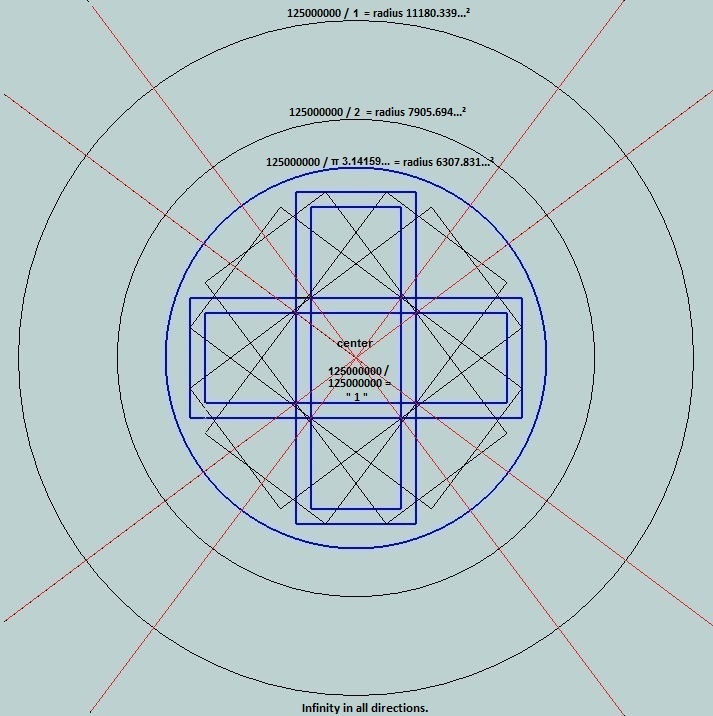Home | Study Links | Poems |

## Square and Circle

Two methods using Pythagorean squares to make the areas of a square and circle approximately equal to pi.
Continued from the previous pageThe three circles represent a spiral.

Method 1: Determining the circle radius by using 3 & 7

5852.34...mm = 34250000
5590.16...mm = 31250000
5220.15...mm = 27250000
+ =.................92750000

92750000 / 7 = 13250000

13250000 x 3 = 39750000 () = Circle radius 6304.76...mm

Square sides of length 11180.33...mm (125000000)

Result: 125000000 / 39750000 = 3.144654088050... (3144654088050 repeats) [4 / 1.272]

4 / 3.144654088050314465408805... = 1.272
4 / 3.144605511029693144278234... = 1.27201964951406896425242...² = ϕ 1.618033988749... (√1.25 + .5)
4 / 3.141592653589793238462643... = 1.27323954473516268615107... (4 / π)Method 2: Junction between square sides of length and red infinity line = circle radius 6250 mm.

Square sides of length 11180.33...mm (125000000)

Result: 125000000 / 39062500 (6250²) = 3.2Circled area " a " enlarged.

Physical limit 3.2Circled area " b " enlarged.

I remembered that Noah's Ark dimensions were 300 x 50 x 30 = 380, so by following the previous method:
380 / 7 = 54.285714... + 6250 = 6304.285714...² = 39744018.36
Then 125000000 / 39744018.36 = 3.145127372
This number is close to the blue π line, and it provided the idea for time lines.

Square and Circle Spiral (If r= 1 then L= √ π)
A Square side of (L)ength 11180.3398874989...²= 125000000
Equivalent:
Squared =
39788735.7729738339...
Multiplied by
π 3.1415926535...
=
125000000The orange triangle represents the 5000 mm by 3125 mm Pyramid covered in the Sun Magic Square, Proof π is by Design and Planet diameter pages, and the blue circle represents π 3.1415926535...From the center working towards π and using seemingly straight linear measurements to the top, or the four corners converging point of the pyramid at "3.2" signifying the visible physical limit, we enter the unseen realm.
Section 3.2 to π 3.141... shows the illusion of straight lines. All are arched.A 3° difference between this Axial tilt method drawing @ 26.565° and Earth's present-day tilt @ 23.5°Ideas on increasing mass where distance contracts and time is slowed.
The measured distance along the red infinity π line from the center is gradual and even, but when the radius is squared and divided with the square’s area number 125000000 to get the numbers on the red infinity line, the numbers increase or slow rapidly depending on the direction of travel. The spaces between the numbers decrease very fast the closer to the center they get, while the numbers increase in size.

This acceleration of numbers towards the center, or core, represents the increasing force of gravity and simultaneous slowing of time, occurring in all forms of substance, ranging from atoms to planets to galaxies. Whereas increasing number spacings away from mass, represents an increasing speed of gravity, which may one day be used as a faster way to communicate and travel. Gravity propagates much faster than the speed of light.
See the increasing and predictable intervals in prime numbers.

So, perhaps the center of mass, an area of no time, is a container for concentrated mass, i.e. light, i.e. information. And, π may identify the c-constant at 186282.397...mps, where light is condensed enough to be called matter, and show that the acceleration of mass creates gravity forces, which creates light waves.Eight Pythagorean 3-4-5 cubical units uniquely arranged, and a pattern for a beautiful diamond cut.

Three of these (eight 3-4-5 cubical units) form a Sphere.
One facing as shown, one oriented horizontally, and one vertical.
It is too difficult for me to draw this, but hopefully you can visualize it.
Fashioned as a Sphere this model reveals an interesting geometric phenomenon.

Plato insisted that squaring the circle (a square equal in area to that of a given circle) can be solved with a straightedge and compass only.

Continue with circling the square.